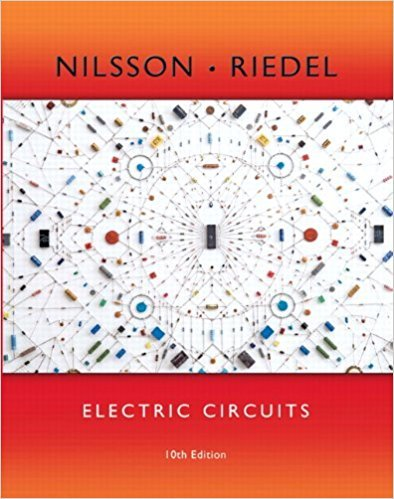×
×

# The 240 V, source in the circuit in Fig. P7.20 is inadvertently short-circuited at itsISBN: 9780133760033 390

## Solution for problem 7.20 Chapter 7

Electric Circuits | 10th Edition

• Textbook Solutions
• 2901 Step-by-step solutions solved by professors and subject experts
• Get 24/7 help from StudySoup virtual teaching assistantsElectric Circuits | 10th Edition

4 5 1 418 Reviews
20
2
Problem 7.20

The 240 V, source in the circuit in Fig. P7.20 is inadvertently short-circuited at its terminals a, b. At the time the fault occurs, the circuit has been in operation for a long time. a) What is the initial value of the current in the short-circuit connection between terminals a, b? b) What is the final value of the current ? c) How many microseconds after the short circuit has occurred is the current in the short equal to 114 A?

Step-by-Step Solution:
Step 1 of 3

Basic Introduction to UNIX/linux Claude Cantin (claude.cantin@nrc.ca) http://www.nrc.ca/imsb/rcsg Research Computing Support Group Information Management Services Branch National Research Council January 31, 2016 2 This page intentionally left blank. 3 This document was produced...

Step 2 of 3

Step 3 of 3

##### ISBN: 9780133760033

This textbook survival guide was created for the textbook: Electric Circuits, edition: 10. The full step-by-step solution to problem: 7.20 from chapter: 7 was answered by , our top Engineering and Tech solution expert on 03/13/18, 07:48PM. Electric Circuits was written by and is associated to the ISBN: 9780133760033. This full solution covers the following key subjects: . This expansive textbook survival guide covers 107 chapters, and 1357 solutions. Since the solution to 7.20 from 7 chapter was answered, more than 214 students have viewed the full step-by-step answer. The answer to “The 240 V, source in the circuit in Fig. P7.20 is inadvertently short-circuited at its terminals a, b. At the time the fault occurs, the circuit has been in operation for a long time. a) What is the initial value of the current in the short-circuit connection between terminals a, b? b) What is the final value of the current ? c) How many microseconds after the short circuit has occurred is the current in the short equal to 114 A?” is broken down into a number of easy to follow steps, and 81 words.

Unlock Textbook Solution